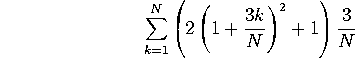M403K Third Midterm Exam Solutions
Exam given April 11, 2002

1. Related rates.

Consider the curve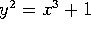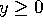.

a) Find the slope of the line that is tangent to the curve at the point (2,3).

Take the derivative of the equation with respect to x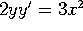, so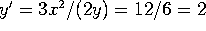.

b) A particle is moving along the curve. Its x-coordinate is increasing at a rate of 10 units/second. How fast is y changing when (x,y)=(2,3)?

There are two reasonably easy solutions. One is to use the result from (a): dy/dt = (dy/dx)(dx/dt) = 2(10) = 20 units/second.

The other method is to start from scratch, and take the derivative of the equation with respect to t: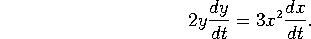Plugging in values of x, y and dx/dt gives 6 (dy/dt)=120, so dy/dt = 20, as before.

Problem 2. L'Hopital's Rule Evaluate the following limits:

a)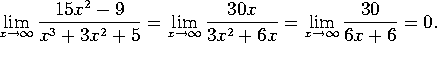b)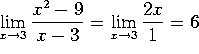.

c)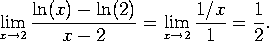d)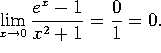L'Hopital's rule does not apply here.

Problem 3. Elasticity of Demand

The demand x for a new toy depends on its price p via the demand equation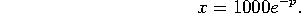a) Compute the elasticity of demand E(p) as a function of p.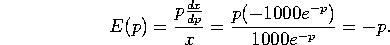b) For what values of p is the demand elastic? For what values of p is the demand inelastic?

When p>1, E<-1 and the system is elastic. [Under these circumstances we should lower the price to increase revenue.]

When p<1, E>-1 and the system is inelastic. [To raise revenue, raise the price].

c) What value of p will maximize revenue?

p=1.

Problem 4. Horse sense

For the first two years of life, a pony's height H(t) grows at a rate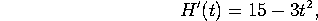(where height is measured in inches and time in years). At age 1, the pony is 45 inches tall.

a) How tall was the pony at birth?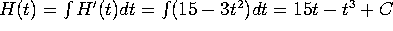. To evaluate the constant, use the fact that H(1)=45, so 45 = 15 - 1 + C, so C=31. Now plug back in to get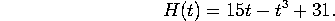So when t was zero, H was 31.

b) How tall will the pony be at age 2?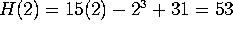inches.

Problem 5. Indefinite integrals.

Evaluate the following integrals:

a)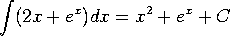b)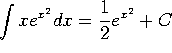. (Integrate by substitution with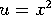.)

c)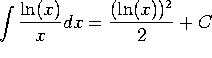. (Integrate by substitution with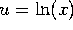.)

d)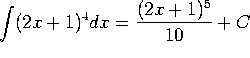. (Integrate by substitution with u=2x+1.)

Problem 6. Area under a curve.

We are interested (OK, OK, your instructor is interested) in finding the area under the curve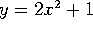between x=1 and x=4.

a) Estimate this area using 3 rectangles. Your final answer should be an explicit number, like 13 or 152.

Each rectangle has width (4-1)/3 = 1. The three rectangles have height f(2), f(3) and f(4), so the estimated total area is f(2)+f(3)+f(4) = 9+19+33 = 61. [If you used the function values at 1, 2 and 3 instead of 2, 3, and 4, I gave full credit. The answer then would be 31]

b) Estimate the area using N rectangles. You can leave your answer as a sum, like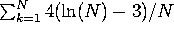(no, that's not the right answer). Everything in the sum needs to be clearly defined, but YOU DO NOT NEED TO SIMPLIFY OR EVALUATE THE SUM.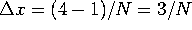and a=1, so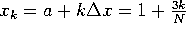. Thus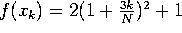, and our estimated area,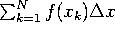, works out to